# excel线性回归，多项式回归的做法和解释

``````p <- 0.5
q <- seq(0,100,1)
y <- p*q
plot(q,y,type='l',col='red',main='线性关系')``````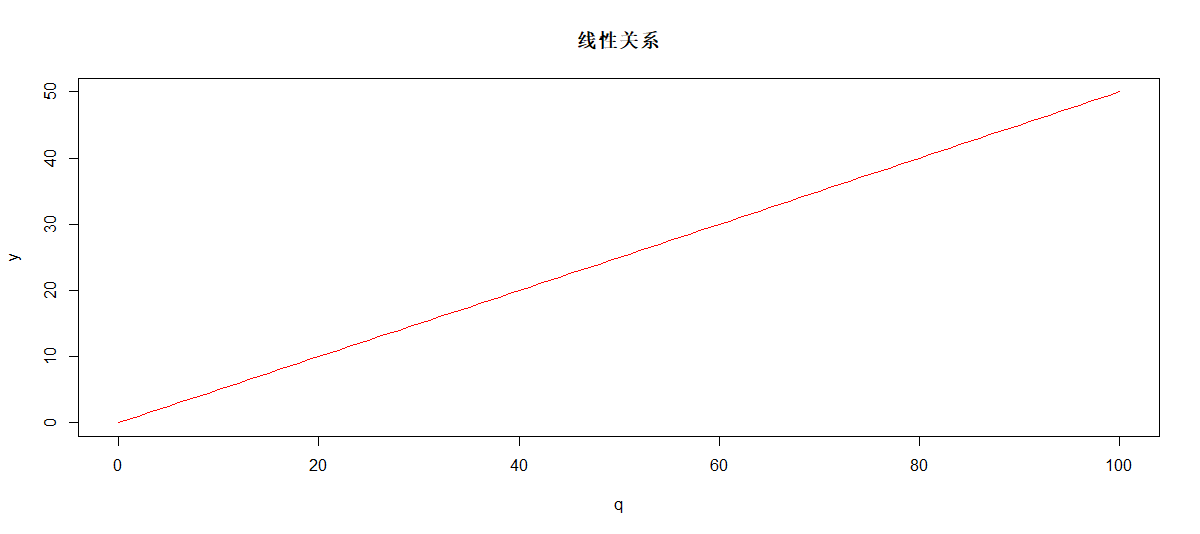``````y <- 450 + p*(q-10)^3
plot(q,y,type='l',col='navy',main='Nonlinear relationship',lwd=3)``````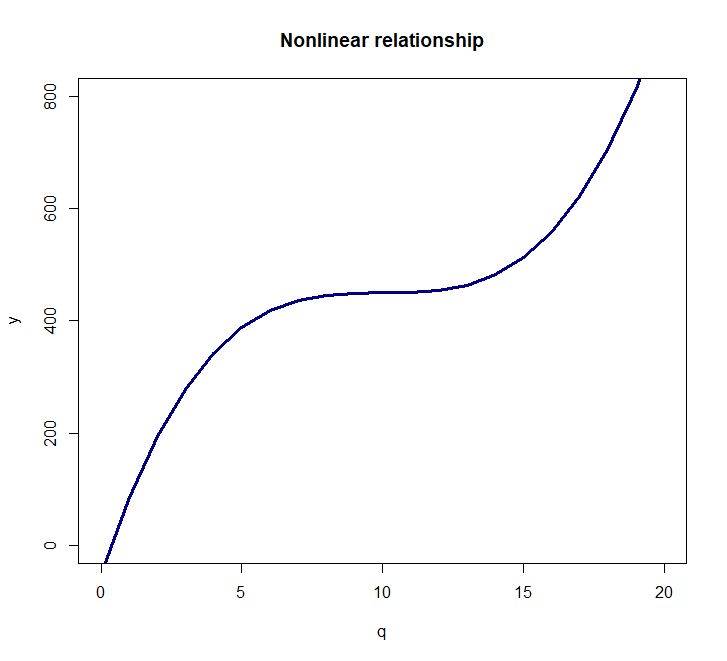# 数据模拟

``````q <- seq(from=0, to=20, by=0.1)
y <- 500 + 0.4 * (q-10)^3
noise <- rnorm(length(q), mean=10, sd=80)
noisy.y <- y + noise``````

``````plot(q,noisy.y,col='deepskyblue4',xlab='q',main='Observed data')
lines(q,y,col='firebrick1',lwd=3)``````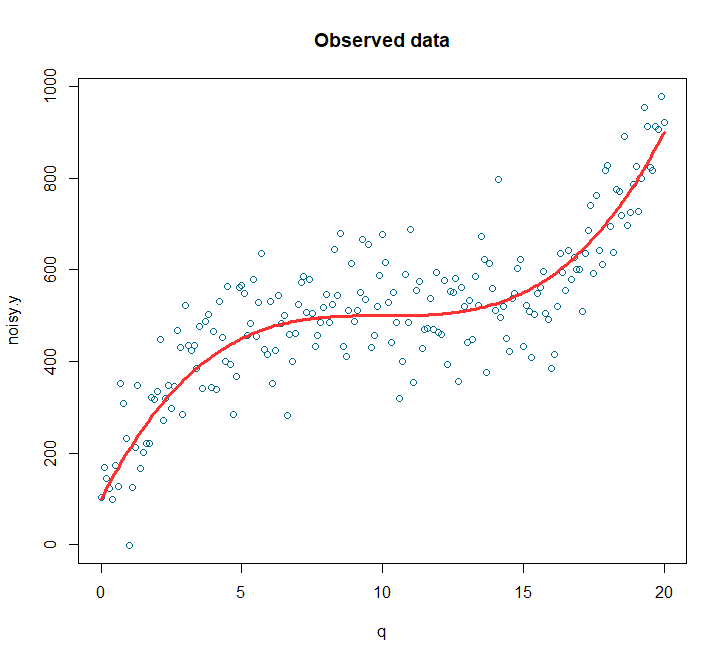# 多项式回归

``````model <- lm(noisy.y ~ poly(q,3))
model <- lm(noisy.y ~ x + I(X^2) + I(X^3))``````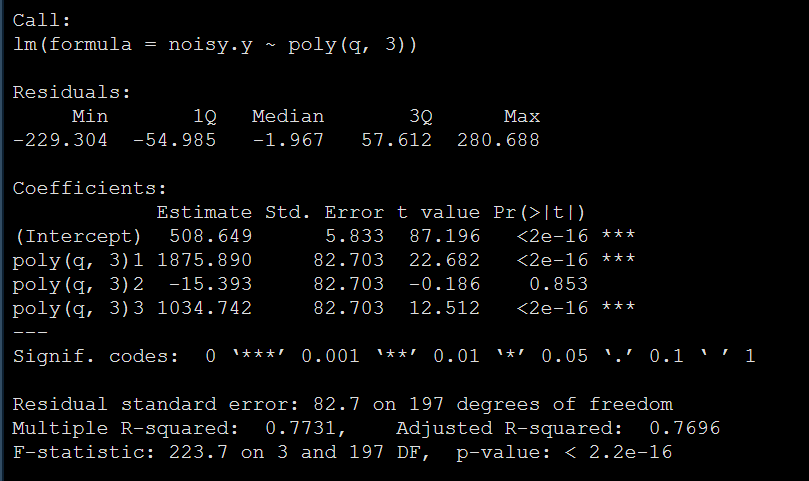``confint(model, level=0.95)``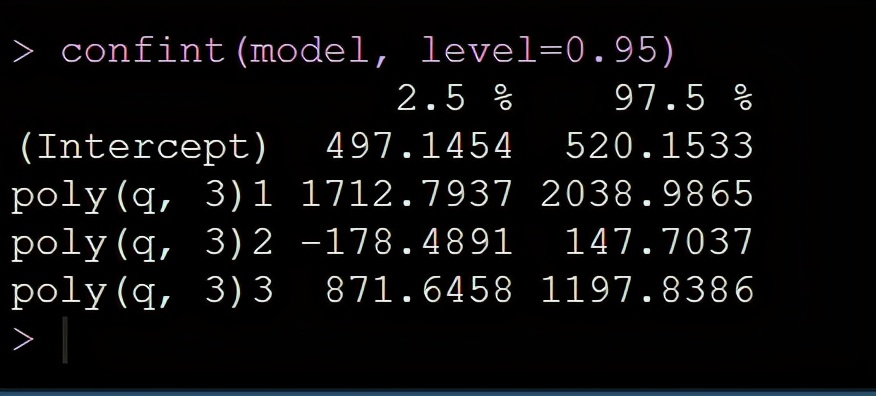``plot(fitted(model),residuals(model))``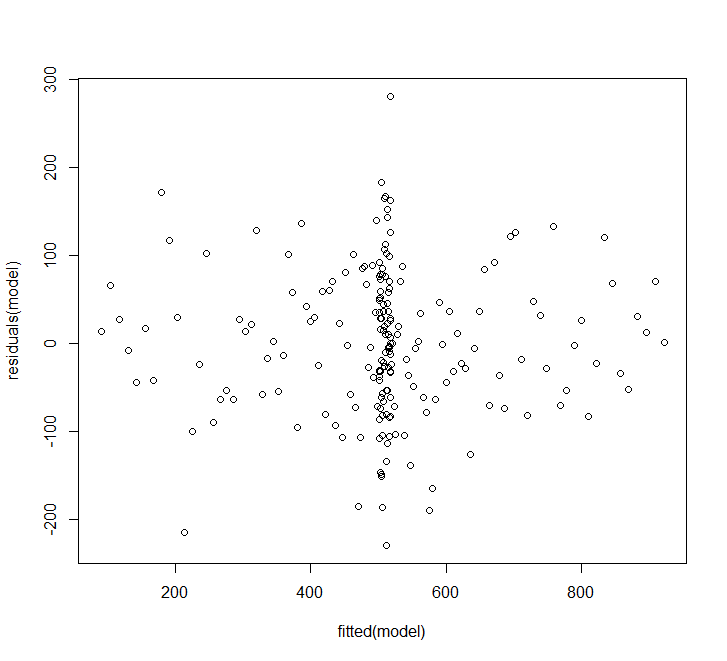``````predicted.intervals <- predict(model,data.frame(x=q),interval='confidence',
level=0.99)``````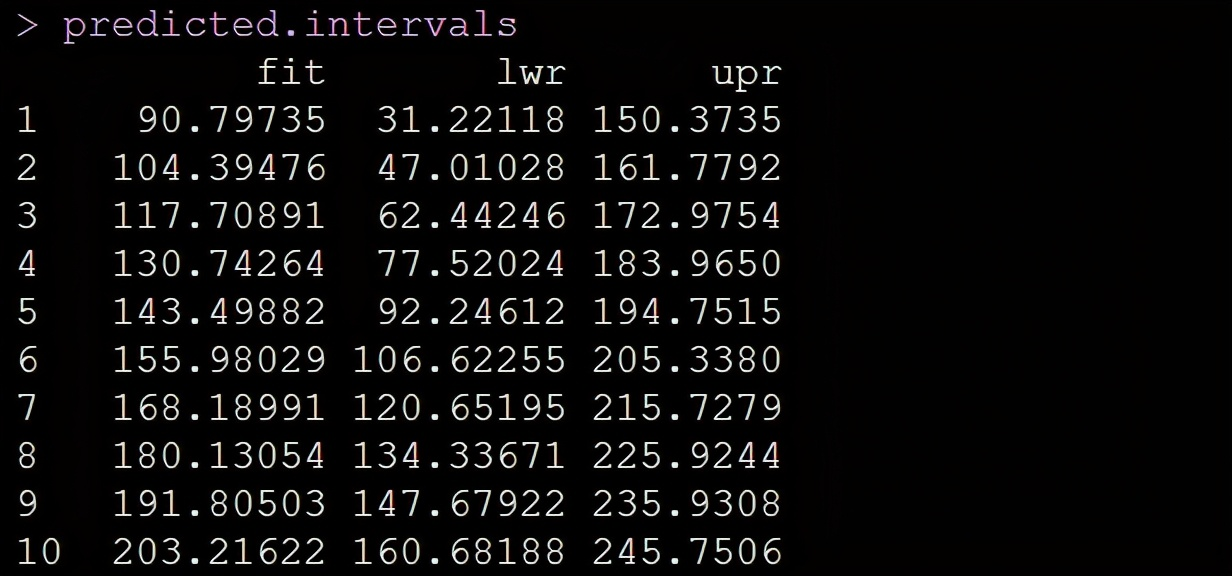``````lines(q,predicted.intervals[,1],col='green',lwd=3)
lines(q,predicted.intervals[,2],col='black',lwd=1)
lines(q,predicted.intervals[,3],col='black',lwd=1)``````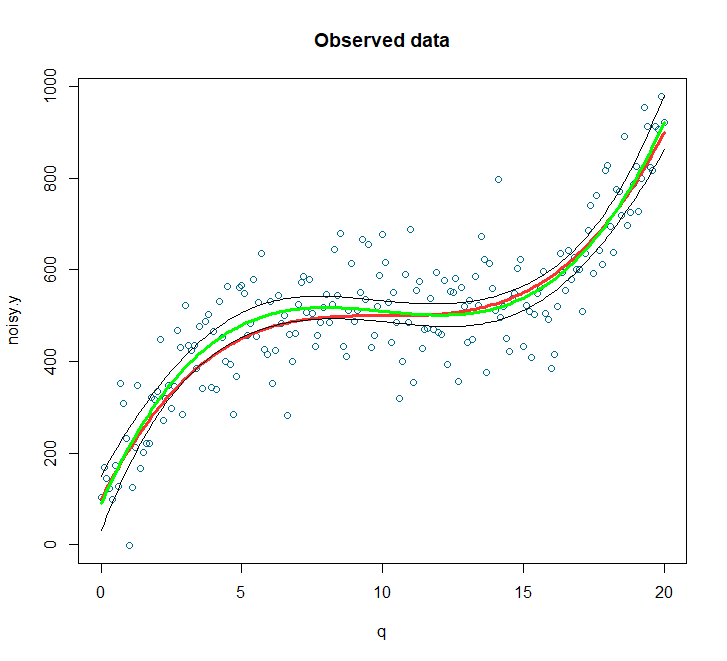# 小结

If you are a student and you are worried about you statistical #Assignments, #Data #Analysis, #Thesis, #reports, #composing, #Quizzes, Exams.. And if you are facing problem in #SPSS, #R-Programming, #Excel, Mplus, then contact me. Because I could provide you the best services for your Data Analysis.

Are you confused with statistical Techniques like z-test, t-test, ANOVA, MANOVA, Regression, Logistic Regression, Chi-Square, Correlation, Association, SEM, multilevel model, mediation and moderation etc. for your Data Analysis...??

Then Contact Me. I will solve your Problem...

# 往期内容：

R数据分析：逻辑斯蒂回归与泊松回归